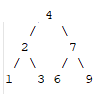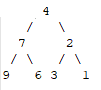### I just announced the new Learn Spring course, focused on the fundamentals of Spring 5 and Spring Boot 2:

>> CHECK OUT THE COURSE

## 1. Overview

Reversing a binary tree is one of the problems that we might be asked to solve during a technical interview.

In this quick tutorial, we’ll see a couple of different ways of solving this problem.

## 2. Binary Tree

A binary tree is a data structure in which each element has at most two children, which are referred to as the left child and the right child. The top element of the tree is the root node, whereas the children are the interior nodes.

However, if a node has no child, it's called a leaf.

Having said that, let's create our object that represents a node:

``````public class TreeNode {

private int value;
private TreeNode rightChild;
private TreeNode leftChild;

// Getters and setters

}``````

Then, let's create our tree that we'll be using in our examples:

``````    TreeNode leaf1 = new TreeNode(1);
TreeNode leaf2 = new TreeNode(3);
TreeNode leaf3 = new TreeNode(6);
TreeNode leaf4 = new TreeNode(9);

TreeNode nodeRight = new TreeNode(7, leaf3, leaf4);
TreeNode nodeLeft = new TreeNode(2, leaf1, leaf2);

TreeNode root = new TreeNode(4, nodeLeft, nodeRight);
``````

In the previous method, we created the following structure:By reversing the tree from left to right, we'll end up having the following structure:## 3. Reversing the Binary Tree

### 3.1. Recursive Method

In the first example, we'll use recursion to reverse the tree.

First of all, we'll call our method using the tree's root, then we'll apply it on the left and the right children respectively until we reach the tree's leaves:

``````public void reverseRecursive(TreeNode treeNode) {
if(treeNode == null) {
return;
}

TreeNode temp = treeNode.getLeftChild();
treeNode.setLeftChild(treeNode.getRightChild());
treeNode.setRightChild(temp);

reverseRecursive(treeNode.getLeftChild());
reverseRecursive(treeNode.getRightChild());
}``````

### 3.2. Iterative Method

In the second example, we'll reverse the tree using an iterative approach. For that, we're going to use a LinkedList, which we initialize with the root of our tree.

Then, for every node we poll from the list, we add its children to that list before we permutate them.

We keep adding and removing from the LinkedList until we reach the tree's leaves:

``````public void reverseIterative(TreeNode treeNode) {

if(treeNode != null) {
}

while(!queue.isEmpty()) {
TreeNode node = queue.poll();
if(node.getLeftChild() != null){
}
if(node.getRightChild() != null){
}

TreeNode temp = node.getLeftChild();
node.setLeftChild(node.getRightChild());
node.setRightChild(temp);
}
}``````

## 4. Conclusion

In this quick article, we explored the two ways of reversing a binary tree. We have started by using a recursive method to reverse it. Then, we ended up using an iterative way to achieve the same result.

The complete source code of these examples and unit test cases can be found over on Github.

### I just announced the new Learn Spring course, focused on the fundamentals of Spring 5 and Spring Boot 2:

>> CHECK OUT THE COURSE
Inline Feedbackscc
1 year ago

Minor nit, method reverseIterative(TreeNode root) does not compile. try renaming parameter root to treeNode. HTHEric Martin
1 year ago